# 全面支持三大主流环境 |百度PaddlePaddle新增Windows环境支持

2019/01/02 20:15

PaddlePaddle作为国内首个深度学习框架，最近发布了更加强大的Fluid1.2版本, 增加了对Windows环境的支持，全面支持了Linux、Mac、 Windows三大环境。 PaddlePaddle在功能完备的基础上，也尽量秉承易学易用的特点，在Windows的安装方面，体现了一键式的特点，大部分情况下，只需要一条简单的命令就可以完成安装。 用户在使用的过程中可能会面对安装和编译方面的问题，下面就从这两个方面来分别说明。

### 1.系统检查

PaddlePaddle目前支持Windows7,8,10系列的专业和企业版本，且只支持64位的操作系统。 PaddlePaddle目前支持的Python版本覆盖了2.7,3.5,3.6,3.7版本，基本上包含了目前主流使用的所有python版本，可以通过如下方式检查操作系统和python版本情况

1. >>>import platformimport platform
2. >>>platform.architecture()
3. ('64bit','WindowsPE') # 64 bits on windows 64 bits'64bit','WindowsPE') # 64 bits on windows 64 bits
4. >>> platform.version()
5. '10.0.17134' # windows 10 # windows 10
6. >>> platform.python_version()
7. '2.7.15' # python 2.7 # python 2.7

### 2.拉取安装包的方式

PaddlePaddle可以选择通过pip命令在线或离线安装，下面展示一下如何离线下载安装包。

PaddlePaddle的安装包已经放到了python官方支持的发布渠道上，打开https://pypi.org/ 网站，按图中所示输入Paddlepaddle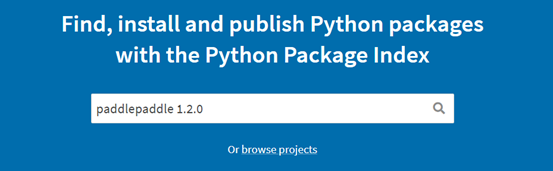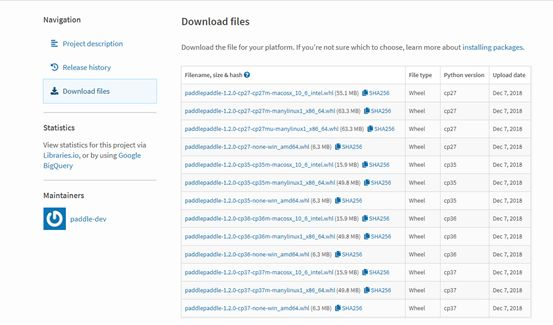### 3.安装过程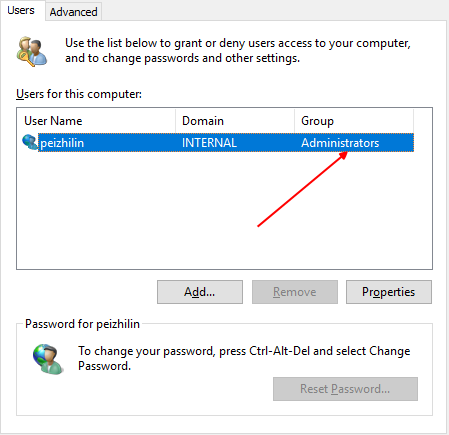### 4.安装完成检查

1. >>> import paddle.fluidimport paddle.fluid
2. >>> print(paddle.__version__)print(paddle.__version__)
3. 1.2.0 # 当前paddle版本.0 # 当前paddle版本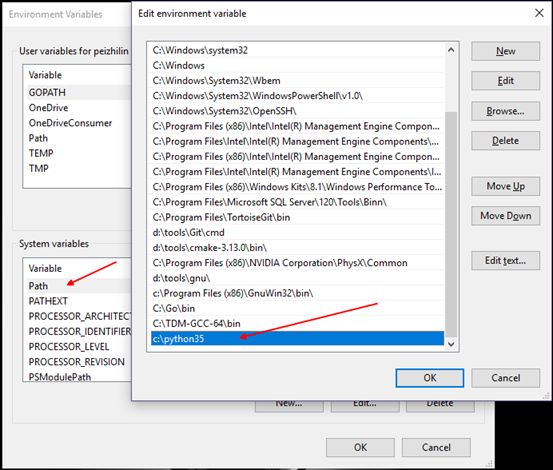### 1.前期准备

#### 2）工具准备

• 2.1 请安装Visual Studio 2015 update3版本。

• 2.2请到 https://cmake.org/download/ 下载cmake 3.0及以上版本 安装

• 2.3请到 https://git-scm.com/download/win 下载git安装

• 2.4请到 https://www.python.org/ 下载python安装，请注意当前支持版本为2.7,3.5,3.6,3.7

*请到 https://pip.pypa.io/en/stable/installing/ 下载pip安装

*请运行 pip install protobuf numpy wheel

### 2. 编译过程

PaddlePaddle的编译过程需要保证网络可用，因为部分依赖包需要通过网络环境获取。 PaddlePaddle编译需要访问 https://github.com/

#### https://github.com/paddlepaddle/paddle 下载源代码，选择 release 1.2 分支，下载zip包或者 通过命令

git clone https://github.com/paddlepaddle/paddle cd paddle git checkout release /1.2

#### 3）运行

1. cmake .. -G "Visual Studio 14 2015 Win64" -DPYTHON_INCLUDE_DIR=${PYTHON_INCLUDE_DIRS} -DPYTHON_LIBRARY=${PYTHON_LIBRARY} -DPYTHON_EXECUTABLE=${PYTHON_EXECUTABLE} -DWITH_FLUID_ONLY=ON -DWITH_GPU=OFF -DWITH_TESTING=OFF -DCMAKE_BUILD_TYPE=Release"Visual Studio 14 2015 Win64" -DPYTHON_INCLUDE_DIR=${PYTHON_INCLUDE_DIRS} -DPYTHON_LIBRARY=${PYTHON_LIBRARY} -DPYTHON_EXECUTABLE=${PYTHON_EXECUTABLE} -DWITH_FLUID_ONLY=ON -DWITH_GPU=OFF -DWITH_TESTING=OFF -DCMAKE_BUILD_TYPE=Release
##### 注释：

a.其中PYTHONINCLUDEDIRS指的是python的include目录，比如 c:\Python35\include\ b.其中PYTHONLIBRARY指定是pythonxx.lib所在的目录，比如c:\Python35\libs\ c.其中PYTHONEXECUTABLE指的是python.exe，比如c:\Python35\ python.exe d.其中DWITHFLUIDONLY=ON指的是paddlepaddle在windows下只支持fluid版本 e.其中WITHGPU=OFF指的是paddlepaddle当前只支持CPU f.其中WITHTESTING=OFF指的是关闭测试 g.其中CMAKEBUILDTYPE=Release指的是只支持Release编译 h.其中-G "Visual Studio 14 2015 Win64"指的是只支持VS2016的64bit编译

### 4） 目录下会生成paddle.sln文件，用Visual Studio 2015打开，选择64位Release模式，开始编译。

#### 2. 常见编译问题

【问】为什么我的paddle.sln文件没有生成？ 【答】请按编译过程要求检查是安装了指定的软件和版本

【问】编译过程中为什么出现ssl一类的网络错误？ 【答】编译过程需要访问网络，请检查系统代理和网络连通情况。

【问】whl文件为什么没有生成？ 【答】请按编译过程要求检查python的变量是否正确设置。

### 1.导入网络

PaddlePaddle在使用方面为了贴合用户需求，尽量做到了将复杂的概念简化，深度学习用户将网络结构会理解为多个层结构的叠加，相对应的，PaddlePaddle也对应的有了层的封装。 在定义网络方面，用户可以统一使用fluid.layers里面定义好的结构，来方面的构建一个神经网络结构，比如

1. # Include libraries.
2. import paddle paddle
3. import paddle.fluid as fluid paddle.fluid as fluid
4. import numpy numpy
5. # Configure the neural network.
6. def net(x, y): net(x, y):
7.     y_predict = fluid.layers.fc(input=x, size=1, act=None)1, act=None)
8.     cost = fluid.layers.square_error_cost(input=y_predict, label=y)
9.     avg_cost = fluid.layers.mean(cost)
10.     return y_predict, avg_costreturn y_predict, avg_cost

### 2. 定义训练和预测函数

1. # Define train function.
2. def train(save_dirname): train(save_dirname):
3.     x = fluid.layers.data(name='x', shape=, dtype='float32')'x', shape=, dtype='float32')
4.     y = fluid.layers.data(name='y', shape=, dtype='float32')'y', shape=, dtype='float32')
5.     y_predict, avg_cost = net(x, y)
6.     sgd_optimizer = fluid.optimizer.SGD(learning_rate=0.001)0.001)
7.     sgd_optimizer.minimize(avg_cost)
8.     train_reader = paddle.batch(
9.         paddle.reader.shuffle(paddle.dataset.uci_housing.train(), buf_size=500),500),
10.         batch_size=20)20)
11.     place = fluid.CPUPlace()CPUPlace()
12.     exe = fluid.Executor(place)Executor(place)
13.     def train_loop(main_program):def train_loop(main_program):
14.         feeder = fluid.DataFeeder(place=place, feed_list=[x, y])DataFeeder(place=place, feed_list=[x, y])
15.         exe.run(fluid.default_startup_program())
16.         PASS_NUM = 10001000
17.         for pass_id in range(PASS_NUM):for pass_id in range(PASS_NUM):
18.             total_loss_pass = 00
19.             for data in train_reader():for data in train_reader():
20.                 avg_loss_value, = exe.run(
21.                     main_program, feed=feeder.feed(data), fetch_list=[avg_cost])
22.                 total_loss_pass += avg_loss_value
23.                 if avg_loss_value < 5.0:if avg_loss_value < 5.0:
24.                     if save_dirname is not None:if save_dirname is not None:
25.                         fluid.io.save_inference_model(
26.                             save_dirname, ['x'], [y_predict], exe)'x'], [y_predict], exe)
27.                     returnreturn
28.             print("Pass %d, total avg cost = %f" % (pass_id, total_loss_pass))print("Pass %d, total avg cost = %f" % (pass_id, total_loss_pass))
29.     train_loop(fluid.default_main_program())
30. # Infer by using provided test data.
31. def infer(save_dirname=None): infer(save_dirname=None):
32.     place = fluid.CPUPlace()CPUPlace()
33.     exe = fluid.Executor(place)Executor(place)
34.     inference_scope = fluid.core.Scope()Scope()
35.     with fluid.scope_guard(inference_scope):with fluid.scope_guard(inference_scope):
36.         [inference_program, feed_target_names, fetch_targets] = (
37.             fluid.io.load_inference_model(save_dirname, exe))
38.         test_reader = paddle.batch(paddle.dataset.uci_housing.test(), batch_size=20)20)
39.         test_data = test_reader().next()next()
40.         test_feat = numpy.array(map(lambda x: x, test_data)).astype("float32")lambda x: x, test_data)).astype("float32")
41.         test_label = numpy.array(map(lambda x: x, test_data)).astype("float32")lambda x: x, test_data)).astype("float32")
42.         results = exe.run(inference_program,
43.                           feed={feed_target_names: numpy.array(test_feat)},0]: numpy.array(test_feat)},
44.                           fetch_list=fetch_targets)
45.         print("infer results: ", results)print("infer results: ", results)
46.         print("ground truth: ", test_label)print("ground truth: ", test_label)

### 3. 执行训练和预测

1. # Run train and infer.
2. if __name__ == "__main__": __name__ == "__main__":
3.     save_dirname = "fit_a_line.inference.model""fit_a_line.inference.model"
4.     train(save_dirname)
5.     infer(save_dirname)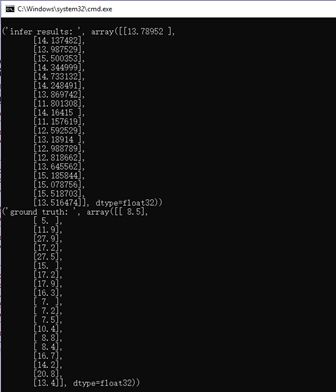0
0 收藏

### 作者的其它热门文章0 评论
0 收藏
0